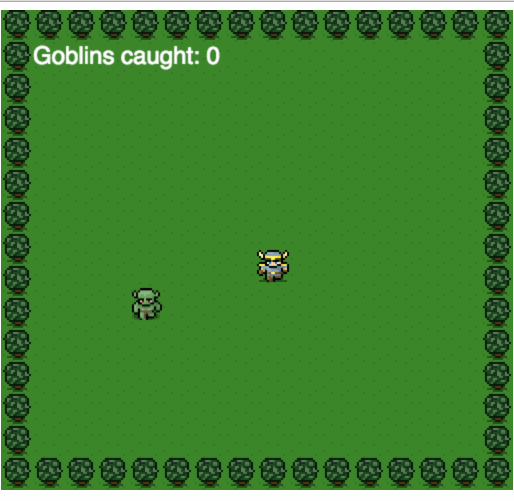# 用HTML5开发一个小游戏

## 先上效果图## 开始之前的准备

1. `game.html`
2. `js/` 里面创建`game.js`
3. `images/` 里面放三张图片，一张背景图片(background.png)，一张英雄图片(hero.png)，一张怪物的图片(monster.png)

`game.html`里面写上以下几行简单的HTML代码：

``````<!DOCTYPE html>
<html lang="en">
<meta charset="utf-8">
<title>Simple Canvas Game</title>
<body>
<script src="js/game.js"></script>
</body>
</html>

``````

## 创建画布

``````var canvas = document.createElement("canvas");
var ctx = canvas.getContext("2d");
canvas.width = 512;
canvas.height = 480;
document.body.appendChild(canvas);

``````

https://developer.mozilla.org/en/canvas_tutorial

## 准备图片

``````// Background image
var bgImage = new Image();
};
bgImage.src = "images/background.png";

// Hero image
var heroImage = new Image();
};
heroImage.src = "images/hero.png";

// Monster image
var monsterImage = new Image();
};
monsterImage.src = "images/monster.png";

``````

``````<img src="images/background.png" >

``````

## 游戏对象

``````var hero = {
speed: 256 // movement in pixels per second
};
var monster = {};
var monstersCaught = 0;

``````

## 处理用户的输入

``````// Handle keyboard controls
var keysDown = {};

keysDown[e.keyCode] = true;
}, false);

delete keysDown[e.keyCode];
}, false);

``````

1. keydown
2. keyup

## 开始一轮游戏

``````// Reset the game when the player catches a monster
var reset = function () {
hero.x = canvas.width / 2;
hero.y = canvas.height / 2;

// Throw the monster somewhere on the screen randomly
monster.x = 32 + (Math.random() * (canvas.width - 64));
monster.y = 32 + (Math.random() * (canvas.height - 64));

};

``````

`reset()`函数用于开始新一轮和游戏，在这个方法里我们将英雄放回画布中心同时将怪物放到一个随机的地方。

## 更新对象

``````var update = function (modifier) {
if (38 in keysDown) { // Player holding up
hero.y -= hero.speed * modifier;
}
if (40 in keysDown) { // Player holding down
hero.y += hero.speed * modifier;
}
if (37 in keysDown) { // Player holding left
hero.x -= hero.speed * modifier;
}
if (39 in keysDown) { // Player holding right
hero.x += hero.speed * modifier;
}

// Are they touching?
if (
hero.x <= (monster.x + 32)
&& monster.x <= (hero.x + 32)
&& hero.y <= (monster.y + 32)
&& monster.y <= (hero.y + 32)
) {

++monstersCaught;
reset();
}

};

``````

`update`函数负责更新游戏的各个对象，会被规律地重复调用。首先它负责检查用户当前按住的是中方向键，然后将英雄往相应方向移动。

`````` if (
hero.x <= (monster.x + 31)
&& monster.x <= (hero.x + 31)
&& hero.y <= (monster.y + 32)
&& monster.y <= (hero.y + 32)
)

``````

## 渲染物体

``````// Draw everything
var render = function () {
ctx.drawImage(bgImage, 0, 0);
}

ctx.drawImage(heroImage, hero.x, hero.y);
}

ctx.drawImage(monsterImage, monster.x, monster.y);
}

// Score
ctx.fillStyle = "rgb(250, 250, 250)";
ctx.font = "24px Helvetica";
ctx.textAlign = "left";
ctx.textBaseline = "top";
ctx.fillText("Goblins caught: " + monstersCaught, 32, 32);
};

``````

## 主循环函数

``````// The main game loop
var main = function () {
var now = Date.now();

var delta = now - then;
//console.log(delta);
update(delta / 1000);
render();

then = now;

// Request to do this again ASAP
requestAnimationFrame(main);
};

``````

## 设置requestAnimationFrame()

``````var w = window;
requestAnimationFrame = w.requestAnimationFrame || w.webkitRequestAnimationFrame || w.msRequestAnimationFrame || w.mozRequestAnimationFrame;

``````

## 最后启动游戏

``````// Let's play this game!
var then = Date.now();
reset();
main();

``````

## 进一步思考

``````var reset = function (x,y) {
hero.x = x;
hero.y = x;
};

``````

``````var update = function (modifier) {

//...other codes

++monstersCaught;
reset(heor.x,hero.y);

};

``````

``````var then = Date.now();
reset(canvas.width / 2,canvas.height / 2);
main();

``````

Hapyy Hacking

16.2k 声望
2k 粉丝
0 条评论